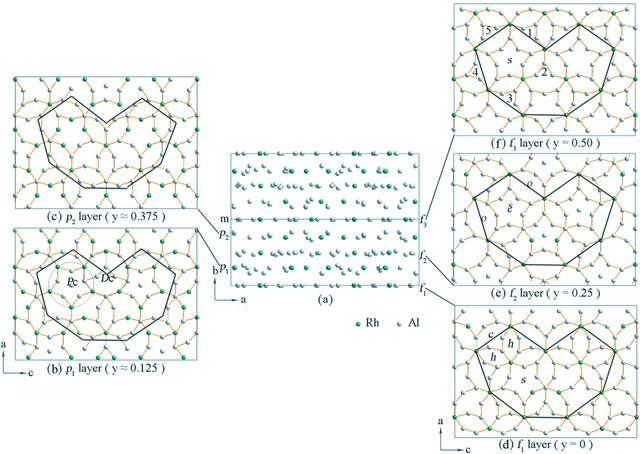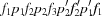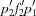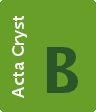disable zoom     view article Figure 6 The atomic structure of ∊16 (a) viewed along the c axis. Eight layers, including four flat layers (f) and four puckered layers (p), are stacked along the b axis. Five independent layers are identified; at y = 0 (f1), ∼ 0.125 (p1), 0.25 (f2), ∼ 0.375 (p2) and 0.5 (f3). The structure can be described as, whereare obtained from the layers p1f2p2 by a mirror symmetry at y = 0.5. (b)–(f) The five independent layers viewed along the b axis. Three basic tilings: squashed hexagon (h), pentagonal star (s) and crown (c) are indicated in (d). A decagonal column (Dc) and a pentagonal column (Pc) are marked in (b). A squashed octagon (o) is marked in (e). A pentagonal star (s) surrounded by five hexagonal columns is marked in (f).STRUCTURAL SCIENCECRYSTAL ENGINEERINGMATERIALS
ISSN: 2052-5206